Select Page

# Unary Operators in Relational Algebra

All those Operators which operate on a single operand are known as unary operators. There are various types of unary operators in relational algebra.

## Types of Unary operators

Unary operators are of three types

1. Projection Operator
2. Selection Operator
3. Rename Operator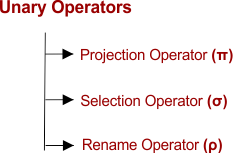### 1. Projection Operator

• Projection Operator (π) displays the columns of a table based on the specified attributes.
• It just works on Columns

Syntax

π<attribute list>(R)

Attribute List contains single or multiple attributes

Example: Consider the following Student relation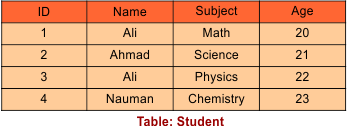Then, we have the Result for Query πID, Name(Student)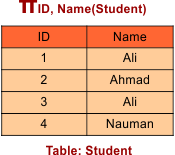Result for Query πName(Student)

Note: This operator removes the redundancy if it is a single column. I.e. The Name “Ali” was twice in the same column (Name) and it removed.But in multiple columns name “Ali” become unique through other tuples as in below examples and does not remove. As First name “Ali” is of age 20 and other is of age 22, so both ”Ali” names are unique.

Result for Query πName, Age(Student)### 2. Selection Operator

• Selection Operator (σ) performs a selection operation.
• It selects those rows or tuples from the table which satisfies the selection condition.
• It works with rows(tuples)

Syntax

σ<selection_condition>(R)

Query1 : Select tuples from a relation “Student” where subject is “Physics”

σsubject = “Physics” (Student)

Result of above query from Student table is given under,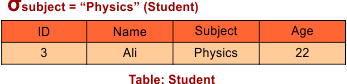Query :Select tuples from a relation “STUDENT” where Name is “Ali” and age is “20”

σName = “Ali” ∧ age = “20” (STUDENT)

Result of above query from Student table is given under,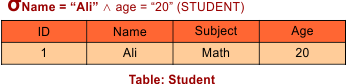#### Important Points of Selection Structure

Point-01:

• Along with selection condition, We may use logical operators (i.e. ! , ∧ , ∨) and relational operators ( i.e. = , ≠ , <=, > etc.).
• Projection operator is used to display the selected tuples.

Point-02:

• Selection operator always selects the entire tuple. So, It cannot select a part of a tuple.

Point-03:

• projection operator does not display the selected tuples.

Point-04:

• Selection operator follows the commutative property i.e. σ X ∧ Y (R) = σ Y ∧ X (R)

Point-05

• Rows selected by selection operation is always less than or equal to the number of rows in the original table.

So,

• Minimum Cardinality = 0
• Maximum Cardinality = |R|

### 3. Rename Operation (ρ)

• To rename relation the rename operation is used which allows us to rename the output relation
• .’Rename’ operation is denoted with rho(ρ).

Syntax:

ρ x (R)

Where the result of current table with expression R is saved with new table name of x.

Note: Through renaming operation we can rename the existing or new output table.

Example: Suppose the following “Student” table#### Case 01: Change the name of given table

To change the name of above table to “STD_Result” Table: Student

Query syntax: ρ STD_Result (Student)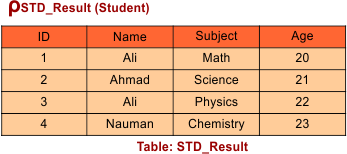#### CASE 02: Change the Name of Output table

Select ID, Name from “Student” table where Subject is “Physics” and rename the output table to STD_Result as well

Query syntax:  ρ STD_Result  (πID, Name (σsubject = “Physics” (Student)))

Output of above query is given below,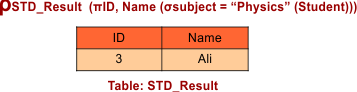#### CASE 03: Rename Output Table and its attributes

Select ID, Name from “Student” table where Subject is “physics” and

• Rename the output table to STD_Result
• Rename attribute ID, Name to STD_ID and STD_Name

Query syntax:  ρ STD_Result (STD_ID, STD_Name)  (πID, Name (σsubject = “Physics” (Student)))

Output of above query given under,Help Other’s By Sharing…